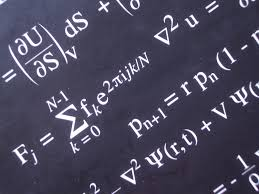Mathematics

The study of all self-consistent structures using numbers, symbols, operations, and logical reasoning. Mathematics is not actually a true science, but instead is employed by the sciences as a tool. All modern fields of science presume at least a familiarity with mathematics. Pure mathematics is an art form that involves the exploration of the elegant properties of numbers, expressions, and geometries according to a well-defined and often elaborate set of rules. Despite its often esoteric nature, pure mathematics does find application in some science fields. Applied mathematics is a more pragmatic study that uses hypotheses based on mathematical techniques in an attempt to resolve scientific problems. Specialties include operations research and statistics.

Views: 30491